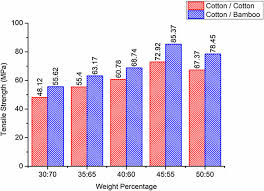## How to Calculate and Solve for Critical Fibre Strength for Composite | Composites

The critical fibre strength for composite is illustrated by the image below.To compute for critical fibre strength for composite, three essential parameters are needed and these parameters are Stress in Matrix at Fibre Failure (σ’m), Fibre Critical Tensile Strength (σ*f) and Volume Fraction of the Fibre (Vf).

The formula for calculating critical fibre strength for composite:

σ*a = σ’m(1 – Vf) + σ*fVf

Where:

σ*a = Critical Fibre Strength for Composite
σ’m = Stress in Matrix at Fibre Failure
σ*f = Fibre Critical Tensile Strength
Vf = Volume Fraction of the Fibre

Let’s solve an example;
Find the critical fibre strength for composite when the stress in matrix at fibre failure is 12, the fibre critical tensile strength is 10 and the volume fraction of the fibre is 9.

This implies that;

σ’m = Stress in Matrix at Fibre Failure = 12
σ*f = Fibre Critical Tensile Strength = 10
Vf = Volume Fraction of the Fibre = 9

σ*a = σ’m(1 – Vf) + σ*fVf
σ*a = 12(1 – 9) + (10)(9)
σ*a = 12(-8) + (90)
σ*a = (-96) + (90)
σ*a = -6

Therefore, the critical fibre strength for composite is -6 Pa.